# Line Of Symmetry - Definition with Examples

The Complete K-5 Math Learning Program Built for Your Child

• 30 Million Kids

Loved by kids and parent worldwide

• 50,000 Schools

Trusted by teachers across schools

• Comprehensive Curriculum

Aligned to Common Core

## Line Of Symmetry Games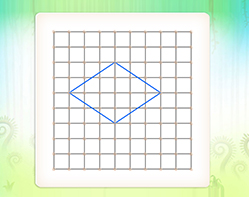Lines of Symmetry

If you fold a figure along a line of symmetry, the two halves exactly fold over each other. Mark lines of symmetry on a variety of closed shapes.

Covers Common Core Curriculum 4.G.3

## What is Line of Symmetry?

We often find symmetry in things around us.
We say there is symmetry when the exact reflection or mirror image of a line, shape or object gets created.

The line of symmetry can be defined as the axis or imaginary line that passes through the center of the shape or object and divides it into identical halves.

Here, for instance, if we fold the figure cut out exactly at the center vertically, its halves will be congruent. The line of the fold is the line of symmetry.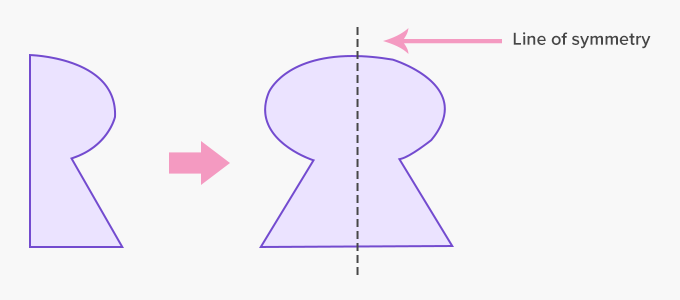Figures or shapes that have exact resemblance to its other part, when divided into two or more equal parts are called symmetrical.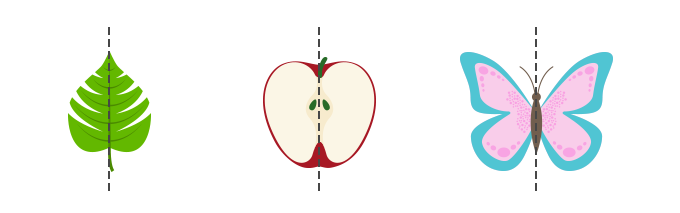The shapes and objects that do not resemble each other when divided into two parts are called asymmetric.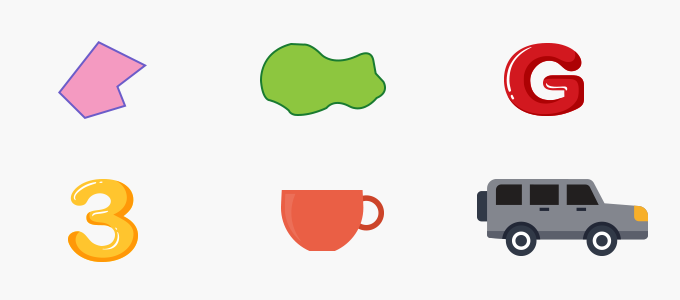Lines of symmetry can be vertical, horizontal or diagonal.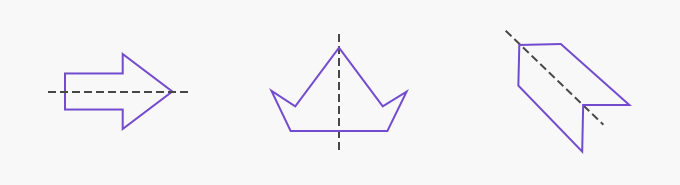The line of symmetry produces reflections that coincide. Here’s how shapes and objects can have multiple lines of symmetry.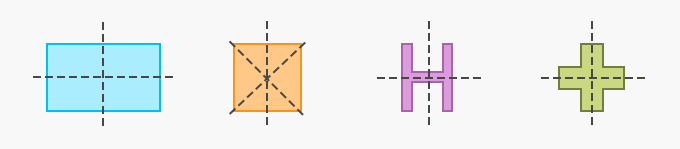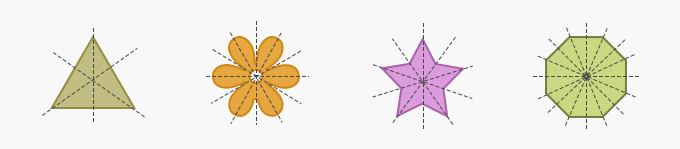Fun Facts The line of symmetry is also called the mirror line or axis of symmetry.  A circle has infinite lines of symmetry.

##Let's do it!

Instead of handing out math worksheets on lines of symmetry to your children, ask them observe objects in their environment that are symmetrical and can be divided by lines of symmetry such a beehive, a flower.

##Related math vocabulary

Won Numerous Awards & Honors